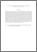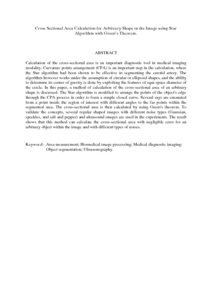# Cross-sectional area calculation for arbitrary shape in the image using star algorithm with Green's theorem

## Citation

Esmaile, Maher Faik and Marhaban, Mohammad Hamiruce and Mahmud, Rozi and Saripan, M. Iqbal (2013) Cross-sectional area calculation for arbitrary shape in the image using star algorithm with Green's theorem. IEEJ Transactions on Electrical and Electronic Engineering, 8 (5). pp. 497-504. ISSN 1931-4973; ESSN: 1931-4981

## Abstract

Calculation of the cross-sectional area is an important diagnostic tool in medical imaging modality. Curvature points arrangement (CPA) is an important step in the calculation, where the Star algorithm had been shown to be effective in segmenting the carotid artery. The algorithm however works under the assumption of circular or ellipsoid shapes, and the ability to determine its center of gravity is done by exploiting the features of equi-space diameter of the circle. In this paper, a method of calculation of the cross-sectional area of an arbitrary shape is discussed. The Star algorithm is modified to arrange the points of the object's edge through the CPA process in order to form a simple closed curve. Several rays are emanated from a point inside the region of interest with different angles to the far points within the segmented area. The cross-sectional area is then calculated by using Green's theorem. To validate the concepts, several regular shaped images with different noise types (Gaussian, speckles, and salt and pepper) and ultrasound images are used in the experiments. The result shows that this method can calculate the cross-sectional area with negligible error for an arbitrary object within the image and with different types of noises.Preview
PDF (Abstract)
Cross Sectional Area Calculation for Arbitrary Shape in the Image using Star Algorithm with Green.pdfView Item